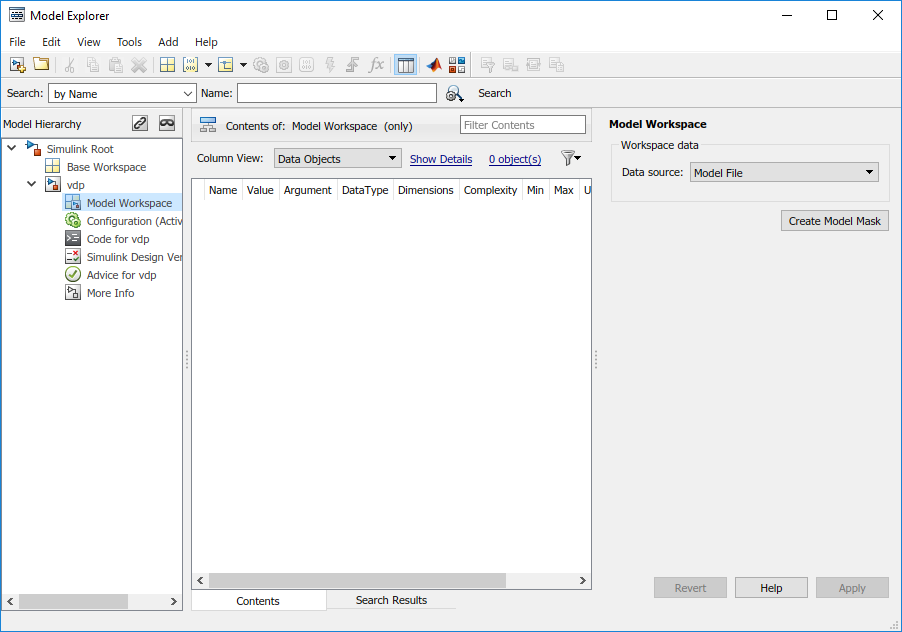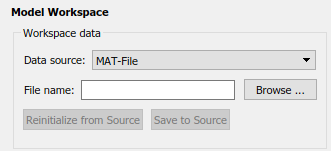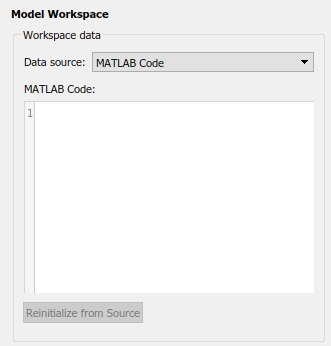## 在模型工作区中指定数据源

• 模型文件，它可以存储静态变量定义。

• 单独的 MAT 文件或 MATLAB® 文件。您可以随时将变量从外部文件重新加载到模型工作区中。

• 您自己的用于创建变量的自定义 MATLAB 代码。您可以将代码保存为模型文件的一部分，并随时重新加载代码。

1. 打开模型资源管理器。在建模选项卡上，点击模型数据编辑器

2. 模型层次结构窗格中，右键点击模型工作区。3. 选择属性菜单项，将打开“模型工作区”对话框。

### 数据源

“模型工作区”对话框中的数据源字段包含工作区的以下数据源选项：

• `模型文件`

指定数据源为模型本身。

• `MAT 文件`

指定数据源为 MAT 文件。选择此选项后，会显示其他控制项（请参阅 MAT 文件和 MATLAB 文件源代码管理）。

• `MATLAB 文件`

指定数据源为 MATLAB 文件。选择此选项后，会显示其他控制项（请参阅 MAT 文件和 MATLAB 文件源代码管理）。

• `MATLAB 代码`

指定数据源是存储在模型文件中的 MATLAB 代码。选择此选项后，会显示其他控制项（请参阅 MATLAB 代码源代码管理）。

### MAT 文件和 MATLAB 文件源代码管理### MATLAB 代码源代码管理